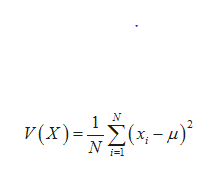what is variance?How do you calculate variance?

Question

what is variance?

How do you calculate variance?

Step 1

Variance:

Variance is the average of squared difference of sample points from their mean. It is a measure of dispersion and it tell how much the data vary from their average. For finding the values of variance, one has to find the difference of the sample point from their mean. The variability from the mean helps to find the spread of the data set from the mean. Moreover, it helps to find the outliers also. As much variability indicates the presence of outliers.

Step 2

The formula for population v...help_outlineImage TranscriptioncloseΝ V (X) - Σ(x- α'. ΝΗ fullscreen

Want to see the full answer?

See Solution

Want to see this answer and more?

Our solutions are written by experts, many with advanced degrees, and available 24/7

See Solution
Tagged in

Other